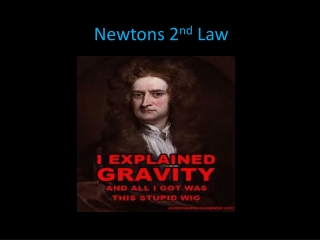DownloadDownload PresentationNewtons 2 nd Law

# Newtons 2 nd Law

Télécharger la présentation## Newtons 2 nd Law

- - - - - - - - - - - - - - - - - - - - - - - - - - - E N D - - - - - - - - - - - - - - - - - - - - - - - - - - -
##### Presentation Transcript

1. Newtons 2nd Law

2. Newton's second law of motion can be formally stated as follows:The acceleration of an object as produced by a net force is directly proportional to the magnitude of the net force, in the same direction as the net force, and inversely proportional to the mass of the object. YIKES!!

3. Instead… • The second law states that the acceleration of an object is dependent upon two variables: • Net Force • Mass Ifnet forceacting on an object theaccelerationof the object the acceleration of the object If the massof an object F and A: Direct relationship M and A: Inverse relationship

4. Newton’s 2nd Law Summarized: The acceleration of an object is directly related to the net force applied and inversely related to the mass of the object. F = M x A

5. Newtons 2nd law can be expressed using this formula… Force = Mass x Acceleration or F= M x A OR... We can use this: F M F a A = m =

6. Rank the following in order of the acceleration of the car from greatest to least. Ties are possible! b a = d c M M A? F a. 2F A? b. 2M 2M F A? c. 2F A? d.

7. Rank the following in order of force required to accelerate the car from greatest to least. Ties are possible: A F? a. M M d b=c a F? A b. 2M 2M F? 2 A c. F? 2 A d.

8. UNITS!! M = F A A = F M F = M x A • These formulas only work if the units are correct: • Mass in KILOGRAMS (kg) • Force in NEWTONS (N) • Acceleration in METERS/SECOND/SECOND (m/s/s or m/s2 )

9. Let’s try a few problems… 1. What net force is required to accelerate a car at a rate of 2 m/s2 if the car has a mass of 3,000 kg? F=_______ m=______ a= _____ 2. What net force is required to accelerate a bowing ball at the same rate of rate of 2 m/s2 if the bowling ball has a mass of 10 kg? F=______ m=______ a=______

10. 3. What is the acceleration of a softball if it has a mass of 0.5 kg and the player throws it with a force of 200 N? F=______ m=______ a=______ 4. What is the acceleration of the 10 kg bowling ball thrown by the same player, throwing with a force of 200N? F=______ m=______ a=______

11. Nooooo…..